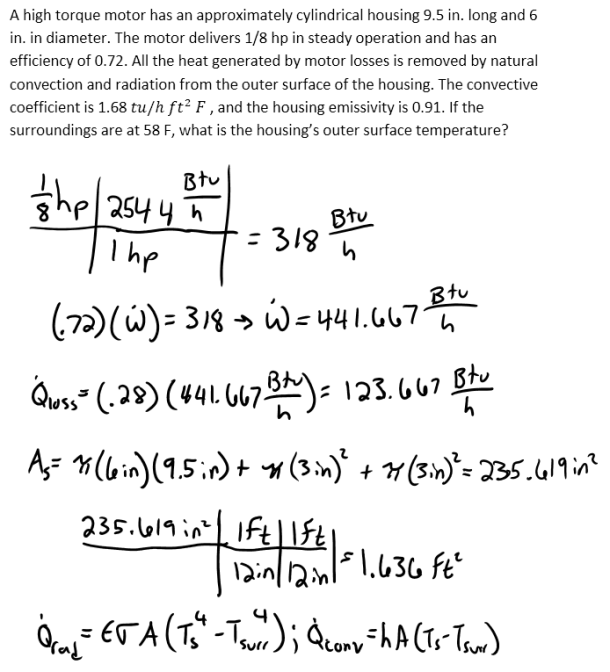heat and mass transfer problems engineering equations heat and mass transfer school homework engineering heat and mass transfer formulas heat and mass transfer solutions to heat and mass transfer problems full solution engineering problem solution heat and mass transfer math problems engineering equations heat and mass transfer school homework engineering solutions to heat and mass transfer formulas heat problems mass problem solutions to transfer problems full solution heat and mass transfer
heat and mass transfer problems engineering equations heat and mass transfer school homework engineering heat and mass transfer formulas heat and mass transfer solutions to heat and mass transfer problems full solution engineering problem solution heat and mass transfer math problems engineering equations heat and mass transfer school homework engineering solutions to heat and mass transfer formulas heat problems mass problem solutions to transfer problems full solution heat and mass transfer
Highalphabet Home Page heat and mass transfer problem solutions Heat and Mass Transfer Page
A high torque motor has an approximately cylindrical housing 9.5 in. long and 6 in. in diameter. The motor delivers 1/8 hp in steady operation and has an efficiency of 0.72. All the heat generated by motor losses is removed by natural convection and radiation from the outer surface of the housing. The convective coefficient is 1.68 tu/h ft^2 F , and the housing emissivity is 0.91. If the surroundings are at 58 F, what is the housing’s outer surface temperature?A high torque motor has an approximately cylindrical housing 9.5 in. long and 6 in. in diameter. The motor delivers 1/8 hp in steady operation and has an efficiency of 0.72. All the heat generated by motor losses is removed by natural convection and radiation from the outer surface of the housing. The convective coefficient is 1.68 tu/h ft^2 F , and the housing emissivity is 0.91. If the surroundings are at 58 F, what is the housing’s outer surface temperature?